2022: SklogWiki celebrates 15 years on-line

Lebwohl-Lasher model

The Lebwohl–Lasher model is a lattice version of the Maier-Saupe mean field model of a nematic liquid crystal . The Lebwohl–Lasher model consists of a cubic lattice occupied by uniaxial nematogenic particles with the pair potential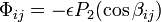$\Phi_{ij} = -\epsilon P_2 (\cos \beta_{ij})$

where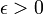$\epsilon > 0$,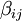$\beta_{ij}$ is the angle between the axes of nearest neighbour particles$i$ and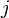$j$, and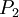$P_2$ is a second order Legendre polynomial.

Isotropic-nematic transition

Fabbri and Zannoni estimated the transition temperature  via a Monte Carlo simulation: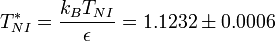$T^*_{NI}= \frac{k_BT_{NI}}{\epsilon}=1.1232 \pm 0.0006$

More recently N. V. Priezjev and Robert A. Pelcovits  used a Monte Carlo cluster algorithm and obtained: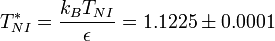$T^*_{NI}= \frac{k_BT_{NI}}{\epsilon}=1.1225 \pm 0.0001$

See also the paper by Zhang et al.  and that of Shekhar et al. .

Confined systems

The Lebwohl–Lasher model has been used to study the effect of confinement in the phase behavior of nematogens 

Planar Lebwohl–Lasher model

The planar Lebwohl–Lasher appears when the lattice considered is two-dimensional. The square lattice is the usual choice for most of the simulation studies. This system exhibits a continuous transition. The ascription of such a transition to the Kosterlitz-Touless type is still under discussion    .

Lattice Gas Lebwohl–Lasher model

This model is the lattice gas version of the Lebwohl–Lasher model. In this case the sites of the lattice can be occupied by particles or empty. The interaction between nearest-neighbour particles is that of the Lebwohl–Lasher model. This model has been studied in .